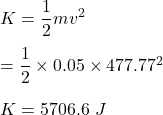A bullet of mass 50 gm is moving with the velocity of 720 km/hr. Calculate the kinetic energy of the bulet​

Question

A bullet of mass 50 gm is moving with the velocity of 720 km/hr. Calculate the kinetic energy of the bulet​

in progress 0
5 months 2021-08-12T23:23:49+00:00 1 Answers 39 views 0

K = 5706.6 J

Explanation:

Given that,

The mass of a bullet, m = 50 g = 0.05 kg

The velocity of the bullet, v = 720 km/hr = 477.77 m/s

We need to find the kinetic energy of the bullet​. The kinetic energy of an object is due to its motion. It can be given by the formula as follows :So, the required kinetic energy of the bullet is equal to 5706.6 J.